### Home > CC2 > Chapter Ch2 > Lesson 2.2.1 > Problem2-39

2-39.

Copy these incomplete number lines. Label the missing numbers on each of them.

1.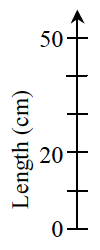Use the information on the number line to determine the value of each segment.

There are $5$ segments that make $50$ cm. How much is each segment?

Each segment is $10$ cm.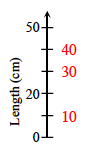1.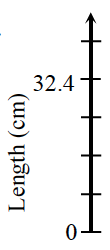See part (a).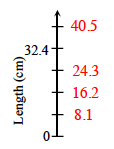1.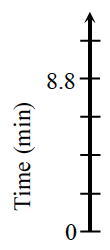Four segments represent $8.8$ minutes. How much is each segment?

1.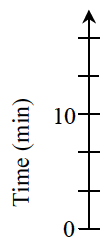See part (a).